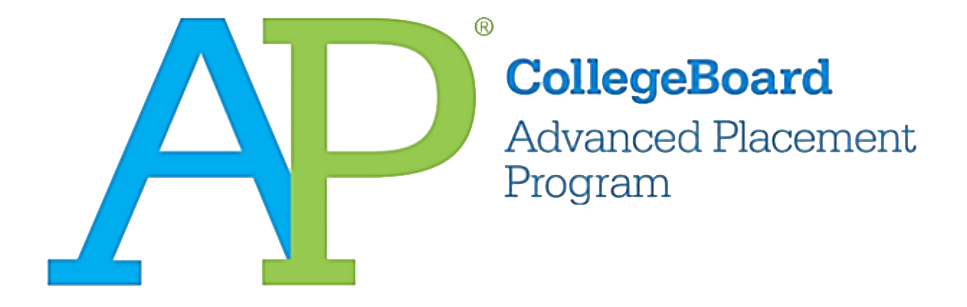# 2016 AP Microeconomics微观经济真题Practice Exam系列之简答题免费下载

### 真题与答案下载### 2016 AP 微观经济模考简答题部分免费下载

3题

#### 部分真题预览：

1）Kohelis Mining is a monopoly and is currently operating at a loss.

1.  Draw a correctly labeled graph and show each of the following.
1. The profit-maximizing quantity, labeled QM
2. The profit-maximizing price, labeled PM
3. The average total cost curve, labeled ATC
4. The allocatively efficient quantity, labeled QA
2. Suppose the government grants Kohelis Mining a lump-sum subsidy such that the firm earns zero economic profit.
1. On your graph from part (a), shade the area of the subsidy.
2. Will the profit-maximizing quantity increase, decrease, or remain the same? Explain.
3. Suppose instead the government provides Kohelis Mining a per-unit subsidy such that the firm earns zero economic profit.
1. Will the profit-maximizing quantity increase, decrease, or remain the same? Explain.
2. Will the price paid by consumers increase, decrease, or remain the same?
4. In this market, will the lump-sum or the per-unit subsidy lead to an increase in the total consumer surplus, or is the change indeterminate? Explain.